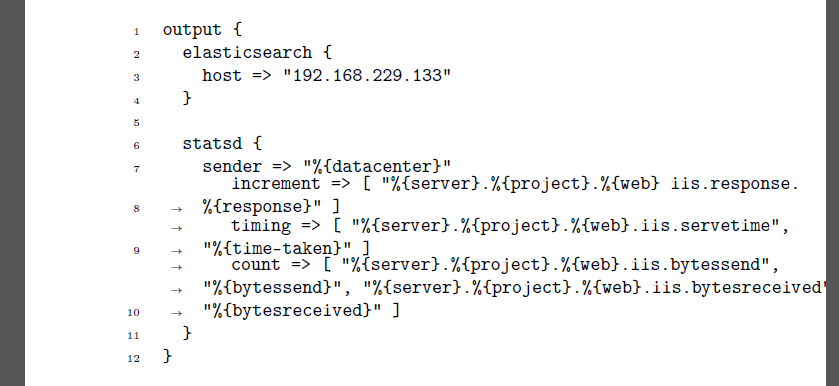# break lines in minted code

Thanks to this question minted is able to break long source code lines.

It is working really fantastic, except in one case: When already indented code gets breaked into two lines, than line two gets a little -> which is nice, but it does not get more intended. Unfortunately the first line gets this little error, too. Unfortunately #2 the line number is omitted, since the first line is already seen as a "breaked line".

An example:

\documentclass[a5paper]{article}
%\pagestyle{empty}
\usepackage[T1]{fontenc}
\usepackage[]{minted}
\usepackage{lineno}
\def\gobble#1{}
\renewcommand\DeleteFile{}
\usepackage{xparse}
\ExplSyntaxOn
\box_new:N \l_fvrb_box
\tl_new:N \l_fvrb_tl

\RenewDocumentCommand \FancyVerbFormatLine { m }
{
\hbox_set:Nn \l_fvrb_box { #1 }
\dim_compare:nNnTF { \box_wd:N \l_fvrb_box }>{ \linewidth }
{%box to big
\tl_set:Nn \l_fvrb_tl { #1 }
\fvrb_use_tl:N \l_fvrb_tl
}
{%box fits
\box_use:N \l_fvrb_box
}
}

\cs_new:Npn \fvrb_use_tl:N  #1
{
\group_begin:
\null\hfill\vbox_set:Nn \l_fvrb_box
{\hsize=\linewidth
\renewcommand\thelinenumber
{
\ifnum\value{linenumber}=1\relax\else
$\rightarrow$
\fi
}
\begin{internallinenumbers}
\hspace*{-2em}\tl_use:N #1
\end{internallinenumbers}
}
\box_use:N \l_fvrb_box
\group_end:
}

\ExplSyntaxOff

\usepackage{etoolbox}
\begin{document}
\begin{minted}[linenos,tabsize=2]{text}
output {
elasticsearch {
host => "192.168.229.133"
}

statsd {
sender => "%{datacenter}"
increment => [ "%{server}.%{project}.%{web}.iis.response. %{response}" ]
timing => [ "%{server}.%{project}.%{web}.iis.servetime", "%{time-taken}" ]
}
}
\end{minted}
\end{document}


The output of itAs you can see, the line numbers and little arrows are a mess. Unfortunately #3 I'm not able to fix this by myself. Is there some one who can help me?

Version 2.1 of minted has breaklines and linenos:

\documentclass[a5paper]{article}
\usepackage[T1]{fontenc}
\usepackage[]{minted}

\begin{document}
\begin{minted}[linenos,tabsize=2,breaklines]{text}
output {
elasticsearch {
host => "192.168.229.133"
}

statsd {
sender => "%{datacenter}"
increment => [ "%{server}.%{project}.%{web}.iis.response. %{response}" ]
timing => [ "%{server}.%{project}.%{web}.iis.servetime", "%{time-taken}" ]